# Sturm theorem

(diff) ← Older revision | Latest revision (diff) | Newer revision → (diff)

If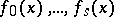(*)

is a Sturm series on the interval,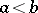, and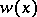is the number of variations of sign in the series (*) at a point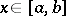(vanishing terms are not taken into consideration), then the number of distinct roots of the function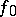on the intervalis equal to the difference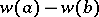.

A Sturm series (or Sturm sequence) is a sequence of real-valued continuous functions (*) onhaving a finite number of roots on this interval, and such that

1)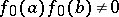;

2)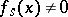on;

3) fromfor some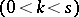and giveninit follows that;

4) fromfor a given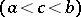it follows that for sufficiently small,This theorem was proved by J.Ch. Sturm , who also proposed the following method of constructing a Sturm series for a polynomialwith real coefficients and without multiple roots: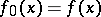,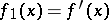, and, if the polynomialsare already constructed, then as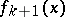one should take minus the remainder occurring in the process of dividingby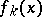. Here,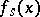will be a non-zero constant.

How to Cite This Entry:
Sturm theorem. Encyclopedia of Mathematics. URL: http://encyclopediaofmath.org/index.php?title=Sturm_theorem&oldid=17606
This article was adapted from an original article by I.V. Proskuryakov (originator), which appeared in Encyclopedia of Mathematics - ISBN 1402006098. See original article